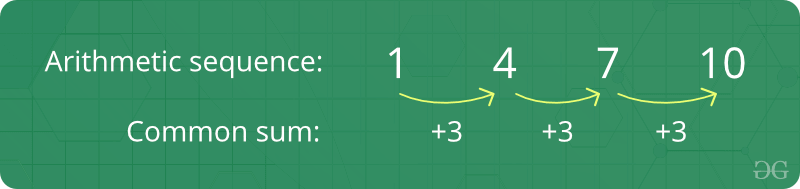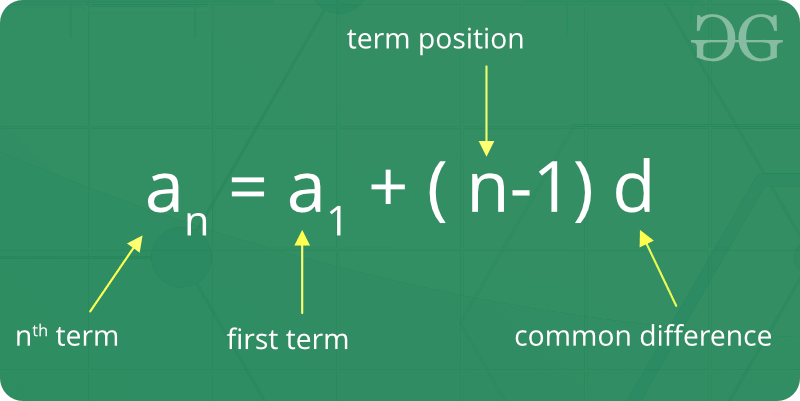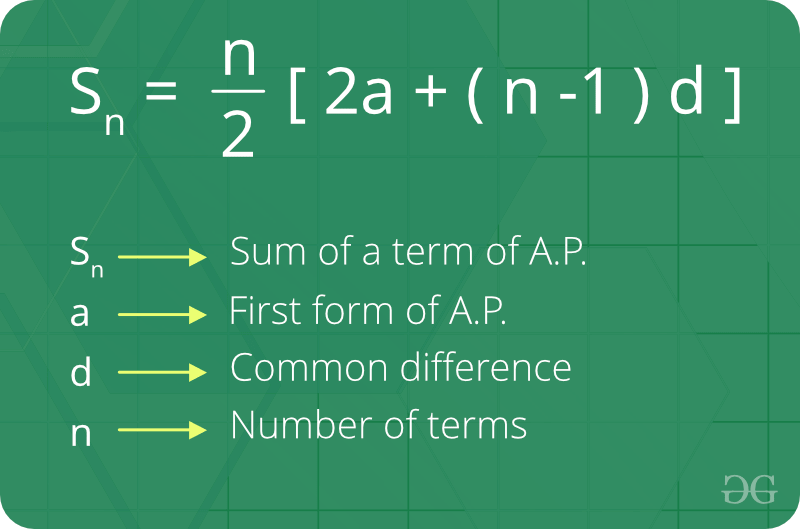Related Articles
Arithmetic Progression
• Last Updated : 10 Dec, 2018

A sequence of numbers is called an Arithmetic progression if the difference between any two consecutive terms is always the same. In simple terms, it means that the next number in the series is calculated by adding a fixed number to the previous number in the series. For example, 2, 4, 6, 8, 10 is an AP because difference between any two consecutive terms in the series (common difference) is same (4 – 2 = 6 – 4 = 8 – 6 = 10 – 8 = 2).Fact about Arithmetic Progression :

1. Initial term: In an arithmetic progression, the first number in the series is called the initial term.
2. Common difference: The value by which consecutive terms increase or decrease is called the common difference.
3. The behavior of the arithmetic progression depends on the common difference d. If the common difference is:positive, then the members (terms) will grow towards positive infinity or negative, then the members (terms) will grow towards negative infinity.

Formula of nth term of an A.P :
If ‘a’ is the initial term and ‘d’ is the common difference.Thus, the explicit formula isFormula of sum of nth term of A.P:How we check whether a series is arithmetic progression or not?

1. Naive solution.
The idea is to sort the given array or series. After sorting, check if differences between consecutive elements are same or not. If all differences are same, Arithmetic Progression is possible.

Below is the implementation of this approach:

## C++

 `// C++ program to check if a given array``// can form arithmetic progression``#include ``using` `namespace` `std;`` ` `// Returns true if a permutation of arr[0..n-1]``// can form arithmetic progression``bool` `checkIsAP(``int` `arr[], ``int` `n)``{``    ``if` `(n == 1)``        ``return` `true``;`` ` `    ``// Sort array``    ``sort(arr, arr + n);`` ` `    ``// After sorting, difference between``    ``// consecutive elements must be same.``    ``int` `d = arr - arr;``    ``for` `(``int` `i = 2; i < n; i++)``        ``if` `(arr[i] - arr[i - 1] != d)``            ``return` `false``;`` ` `    ``return` `true``;``}`` ` `// Driven Program``int` `main()``{``    ``int` `arr[] = { 20, 15, 5, 0, 10 };``    ``int` `n = ``sizeof``(arr) / ``sizeof``(arr);`` ` `    ``(checkIsAP(arr, n)) ? (cout << ``"Yes"` `<< endl) : (cout << ``"No"` `<< endl);`` ` `    ``return` `0;``}`

## Java

 `// Java program to check if a given array``// can form arithmetic progression``import` `java.util.Arrays;`` ` `class` `GFG {`` ` `    ``// Returns true if a permutation of``    ``// arr[0..n-1] can form arithmetic``    ``// progression``    ``static` `boolean` `checkIsAP(``int` `arr[], ``int` `n)``    ``{``        ``if` `(n == ``1``)``            ``return` `true``;`` ` `        ``// Sort array``        ``Arrays.sort(arr);`` ` `        ``// After sorting, difference between``        ``// consecutive elements must be same.``        ``int` `d = arr[``1``] - arr[``0``];``        ``for` `(``int` `i = ``2``; i < n; i++)``            ``if` `(arr[i] - arr[i - ``1``] != d)``                ``return` `false``;`` ` `        ``return` `true``;``    ``}`` ` `    ``// driver code``    ``public` `static` `void` `main(String[] args)``    ``{``        ``int` `arr[] = { ``20``, ``15``, ``5``, ``0``, ``10` `};``        ``int` `n = arr.length;`` ` `        ``if` `(checkIsAP(arr, n))``            ``System.out.println(``"Yes"``);``        ``else``            ``System.out.println(``"No"``);``    ``}``}`` ` `// This code is contributed by Anant Agarwal.`

## Python3

 `# Python3 program to check if a given ``# array can form arithmetic progression`` ` `# Returns true if a permutation of arr[0..n-1]``# can form arithmetic progression``def` `checkIsAP(arr, n):``    ``if` `(n ``=``=` `1``): ``return` `True`` ` `    ``# Sort array``    ``arr.sort()`` ` `    ``# After sorting, difference between``    ``# consecutive elements must be same.``    ``d ``=` `arr[``1``] ``-` `arr[``0``]``    ``for` `i ``in` `range``(``2``, n):``        ``if` `(arr[i] ``-` `arr[i``-``1``] !``=` `d):``            ``return` `False`` ` `    ``return` `True`` ` `# Driver code``arr ``=` `[ ``20``, ``15``, ``5``, ``0``, ``10` `]``n ``=` `len``(arr)``print``(``"Yes"``) ``if``(checkIsAP(arr, n)) ``else` `print``(``"No"``)`` ` `# This code is contributed by Anant Agarwal.`

## C#

 `// C# program to check if a given array``// can form arithmetic progression``using` `System;`` ` `class` `GFG {`` ` `    ``// Returns true if a permutation of``    ``// arr[0..n-1] can form arithmetic``    ``// progression``    ``static` `bool` `checkIsAP(``int``[] arr, ``int` `n)``    ``{``        ``if` `(n == 1)``            ``return` `true``;`` ` `        ``// Sort array``        ``Array.Sort(arr);`` ` `        ``// After sorting, difference between``        ``// consecutive elements must be same.``        ``int` `d = arr - arr;``        ``for` `(``int` `i = 2; i < n; i++)``            ``if` `(arr[i] - arr[i - 1] != d)``                ``return` `false``;`` ` `        ``return` `true``;``    ``}`` ` `    ``// Driver Code``    ``public` `static` `void` `Main()``    ``{``        ``int``[] arr = { 20, 15, 5, 0, 10 };``        ``int` `n = arr.Length;`` ` `        ``if` `(checkIsAP(arr, n))``            ``Console.WriteLine(``"Yes"``);``        ``else``            ``Console.WriteLine(``"No"``);``    ``}``}`` ` `// This code is contributed by vt_m.`

## PHP

 ``

Output:
```Yes
```

Time Complexity: O(n Log n).

2. Efficient solutions

Basic Program related to Arithmetic Progression

More problems related to Arithmetic Progression

Attention reader! Don’t stop learning now. Get hold of all the important DSA concepts with the DSA Self Paced Course at a student-friendly price and become industry ready.

My Personal Notes arrow_drop_up